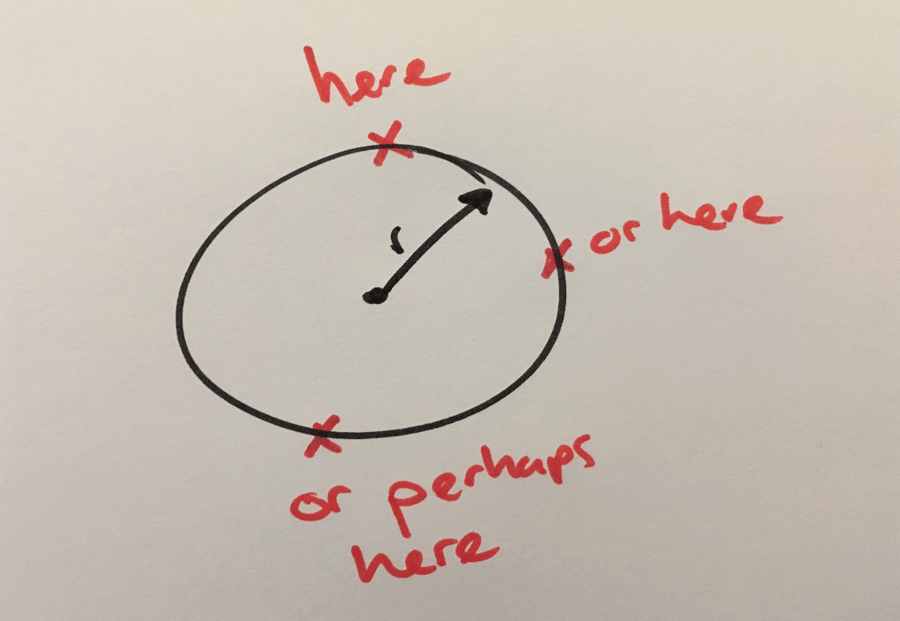Would you like to calculate a random point on a circle edge 3D space like a planet orbit around the sun? This artice shows how to calculate a random point in a circle, put it on the circle edge and convert it from 2D to 3D.

# Calculate a random point on a circle edge in Unity3D.The `Random` class in Unity3D provides the method `insideUnitCircle` that returns a random Vector2 inside a circle with radius = 1. By calling `normalized`, the Vector length (or magnitude) will be increased to 1. That effectively puts the random point on the edge of the circle.

The `RandomPointOnCircleEdge` function returns the random point as a Vector3 where y=0.

``````private Vector3 RandomPointOnCircleEdge(float radius)
{
var vector2 = Random.insideUnitCircle.normalized * radius;
return new Vector3(vector2.x, 0, vector2.y);
}
``````
Written by Loek van den Ouweland on 2017-10-01.
Questions regarding this artice? You can send them to the address below.